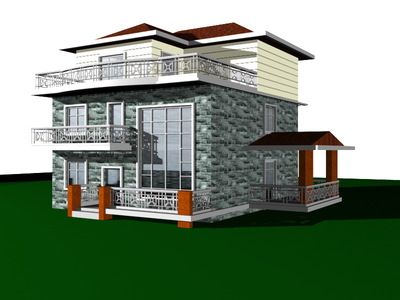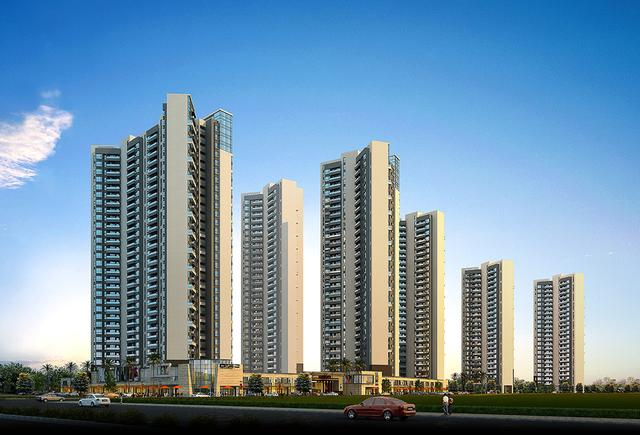# 设某建筑物按设计要求使用寿命超过50年的概率为0.8，超过60年的概率为0.7

P(B'/A)=1-P(B/A)=1-P(AB)/P(A)
=1-P(B)/P(A)=1-0.7/0.8
=1/8

P(B'/A)=1-P(B/A)=1-P(AB)/P(A)
=1-P(B)/P(A)=1-0.7/0.8
=1/8